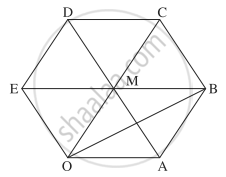# OABCDE is a regular hexagon. The points A and B have position vectors aa¯ and bb¯ respectively referred to the origin O. Find, in terms of aa¯ and bb¯ the position vectors of C, D and E. - Mathematics and Statistics

Sum

OABCDE is a regular hexagon. The points A and B have position vectors bar"a" and bar"b" respectively referred to the origin O. Find, in terms of bar"a" and bar"b" the position vectors of C, D and E.

#### SolutionGiven: bar"OA" = bar"a", bar"OB" = bar"b"

Let AD, BE, OC meet at M.

Then M bisects AD, BE, OC.

bar"AB" = bar"AO" + bar"OB"

= - bar"OA" + bar"OB"

= - bar"a" + bar"b" = bar"b" - bar"a"

∵ OABM is a parallelogram

∴ bar"OM" = bar"AB" = bar"b" - bar"a"

bar"OC" = 2bar"OM" = 2(bar"b" - bar"a") = 2bar"b" - 2bar"a"

bar"OD" = bar"OC" + bar"CD"

= bar"OC" - bar"DC"

= bar"OC" - bar"OA"    ...[∵ OA = DC and OA || DC]

= 2bar"b" - 2bar"a" - bar"a"

= 2bar"b" - 3bar"a"

bar"OE" = bar"OM" + bar"ME"

= (bar"b" - bar"a") - bar"EM"

= bar"b" - bar"a" - bar"a"   ....[∵ EM = OA and EM || OA]

= bar"b" - 2bar"a"

Hence, the position vectors of C, D and E are 2bar"b" - 2bar"a", 2bar"b" - 3bar"a"  "and"  bar"b" - 2bar"a" respectively.

#### Notes

[Note: Answer to bar"OC" in the textbook is incorrect.]

Concept: Vectors and Their Types
Is there an error in this question or solution?